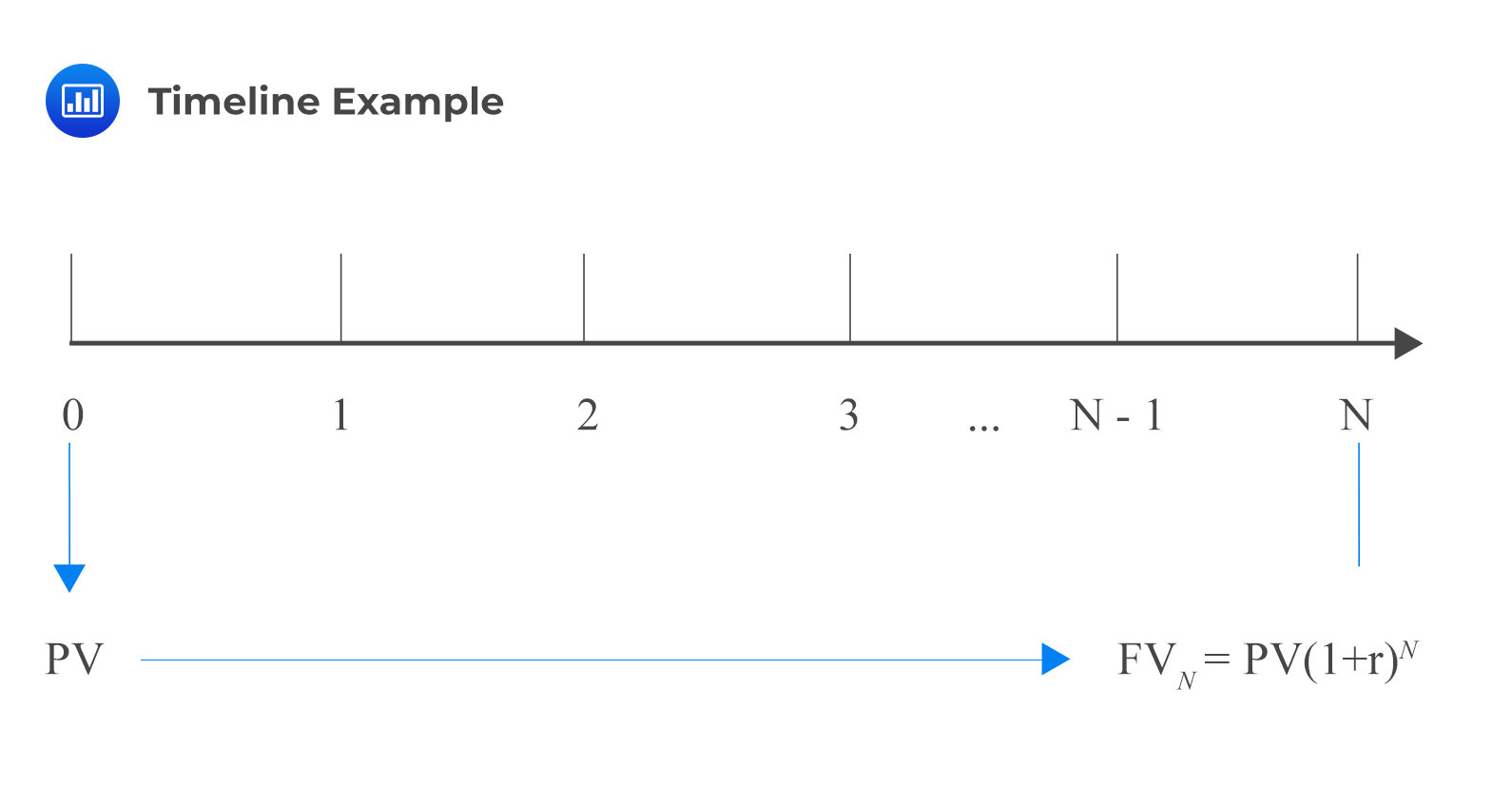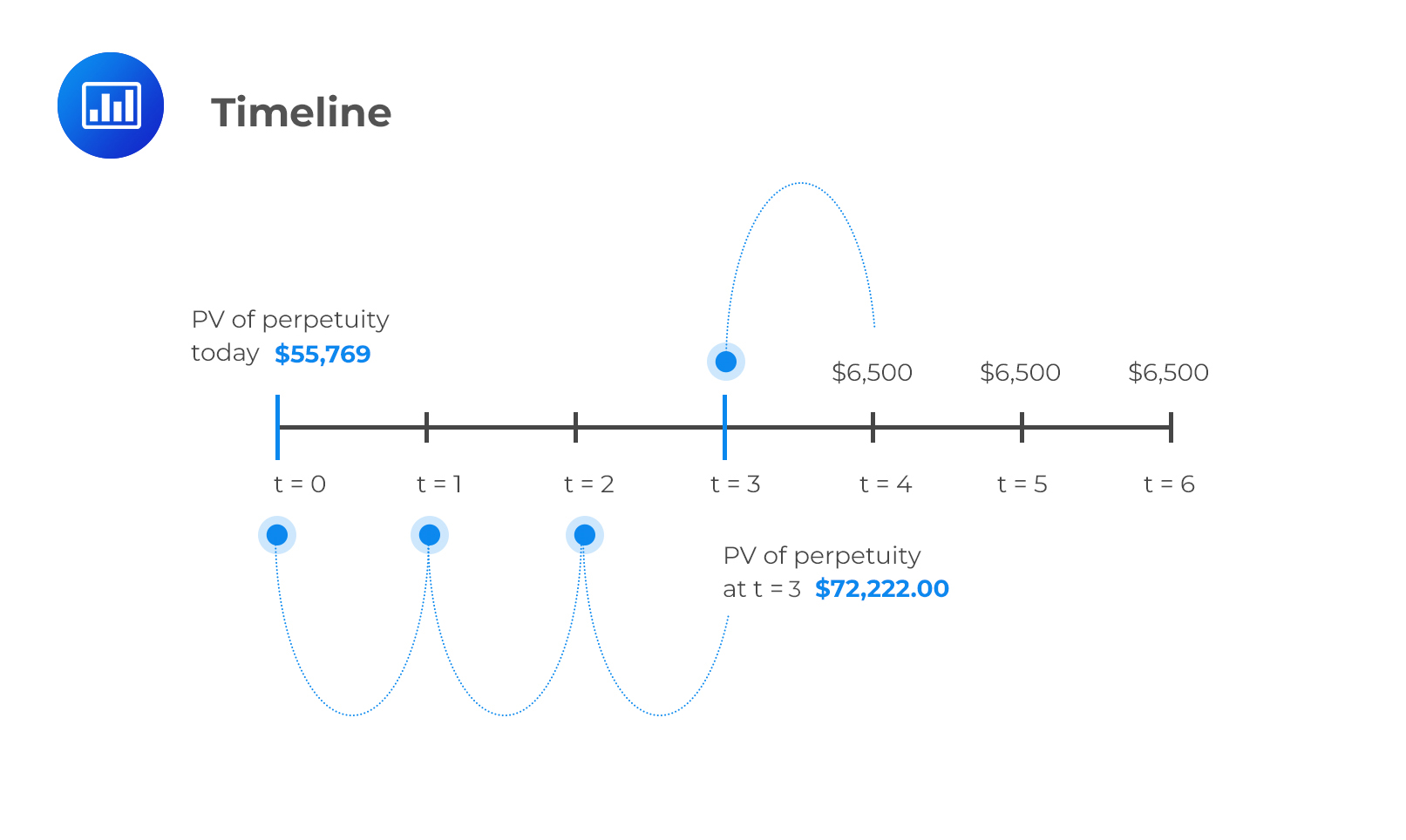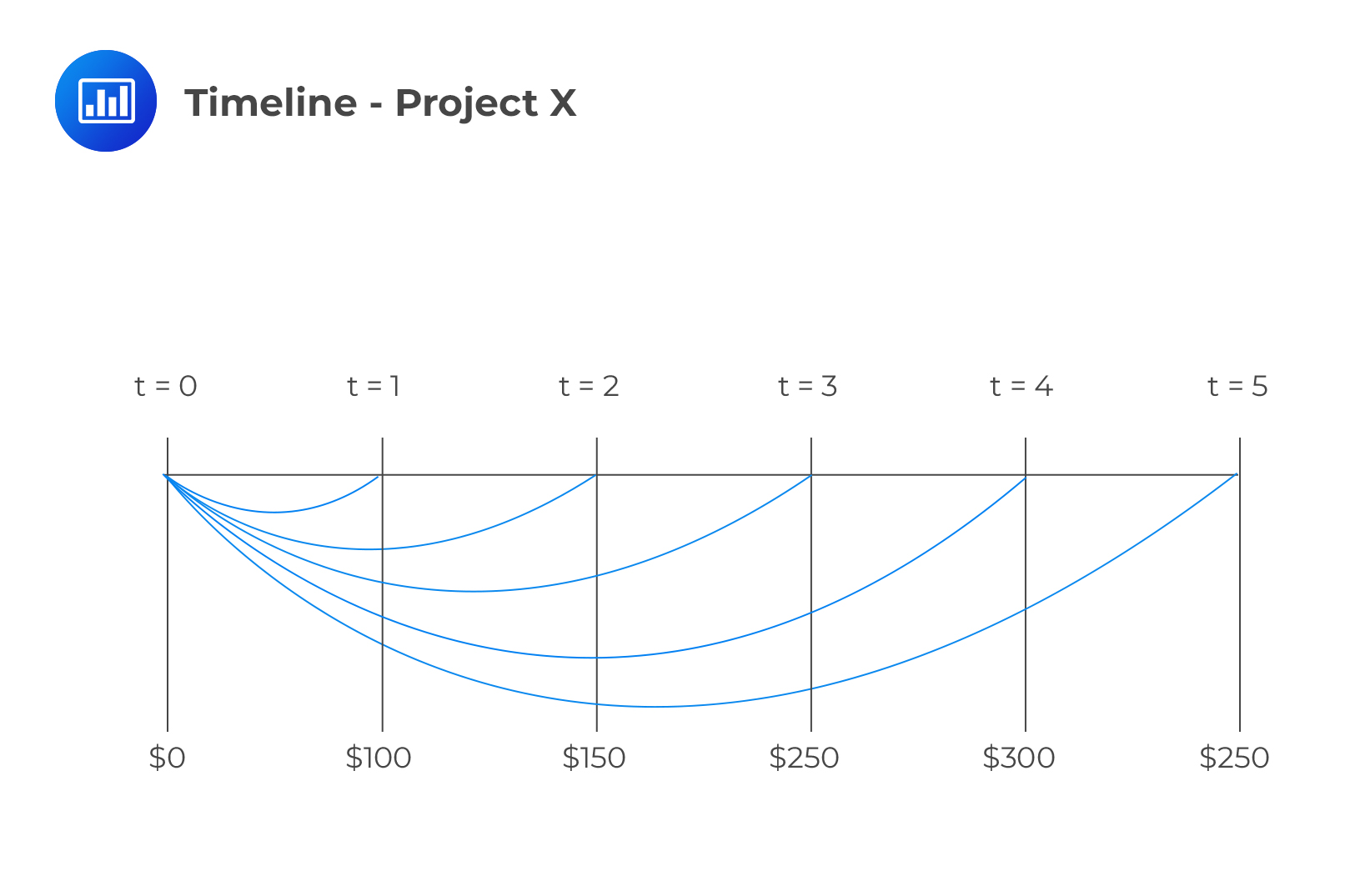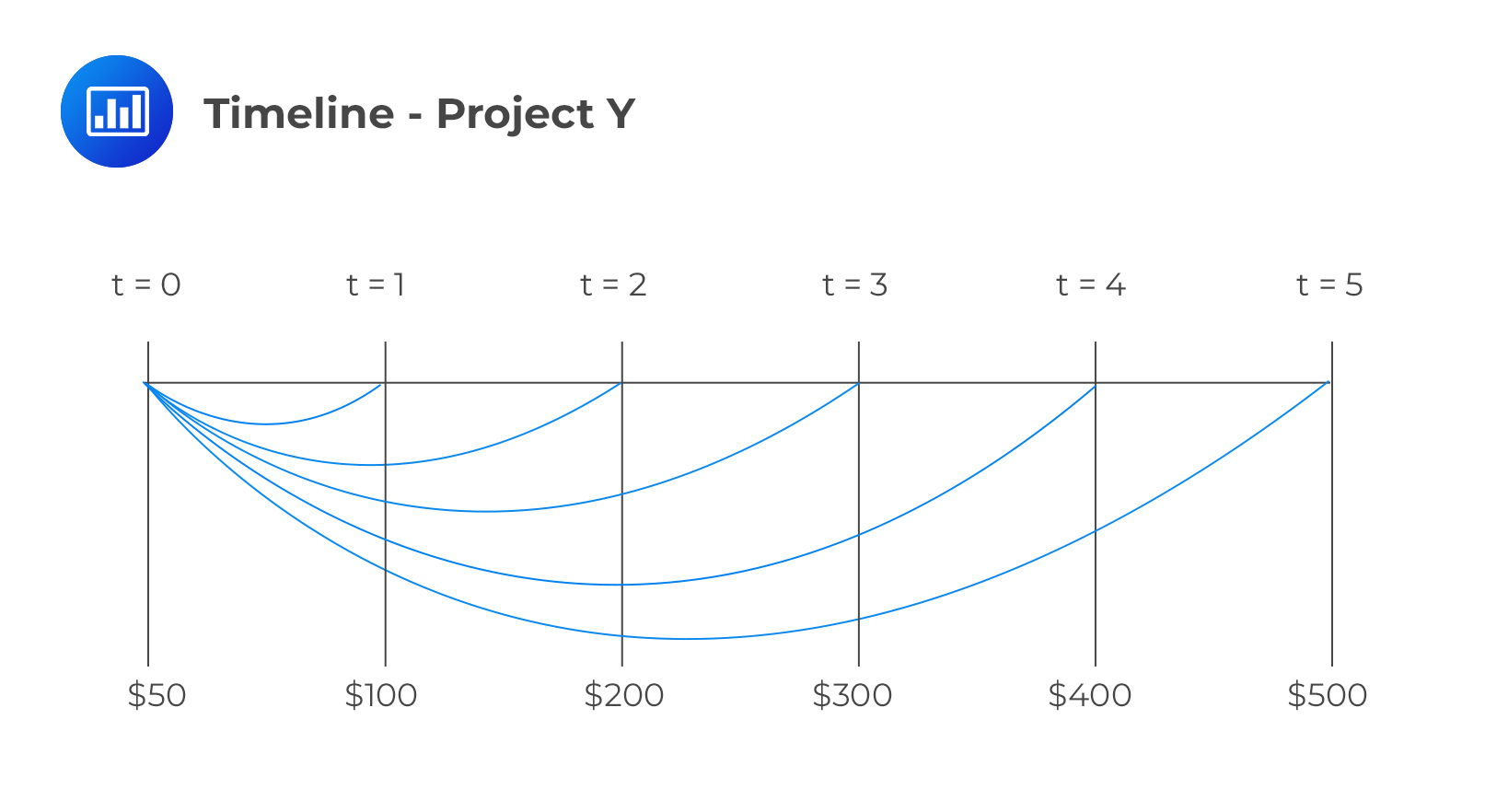# Using a Timeline to Model Cash Flows and Solve Time Value of Money Problems

A timeline is a physical illustration of the amounts and timing of cash flows associated with an investment project. Cash flows that are regular and of equal amounts can be modeled as annuities. In such exam problems, all you have to do is apply the standard annuity formulas. It is even more convenient if you use the approved calculators to do most of the work. However, it may get a little tricky if the cash flows are irregular, unequal, or both. It is essential to draw a timeline to help you understand the problem.

Remember that the general formula that relates the present value and the future value of an investment is given by:

$$FV_{N}=PV\left(1+r\right)^{N}$$

Where

PV = present value of the investment
FVN = future value of the investment N periods from today
r = rate of interest per period

We can represent this in a timeline:A timeline visualizes the compatibility between the time units and the corresponding interest rate per unit time. In a particular timeline, a time index t represents a particular point in time, a specified number of periods from today. Therefore, the present value is the investment amount today (t=0). We can use this amount to calculate the future value (t=N). Alternatively, we can use the future value to calculate the present value.

The above argument can be written in terms of the present value. That is:

$$PV=FV_{N}\left(1+r\right)^{-N}$$

### Example: Applying Timeline to Model Cashflows

An investor receives a series of payments, each amounting to $6,500, set to be received in perpetuity. Payments are to be made at the end of each year, starting at the end of year 4. If the discount rate is 9%, then what is the present value of the perpetuity at t=0? Solution We should draw a timeline to understand the problem better.Here, we can see that the investor is receiving$6,500 in perpetuity (lasts forever). Note that the PV of a PV is given by:

$$\text{PV of a perpetuity} =\frac {C}{r}$$

So that in this case:

$$\text{PV}=\frac {6,500}{9\%} = 72,222$$

This is the value of the perpetuity at t=3, so we need to discount it 3 more periods to get the value at t=0. Here is the formula to use:

$$PV=FV_{N}\left(1+r\right)^{-N}$$

PV at time zero =$$\frac {72,222}{{(1+0.09)}^3} = 55,769$$

## Why is the Use of a Timeline Recommended?

There are many instances in real life when cash flows are uneven. A good case in point is pension contributions which vary with age. In such problems, it’s not possible to apply one of the basic time value formulae. You’re advised to draw a timeline even if the question appears quite straightforward. The timeline will help you understand the question structure better. A timeline also helps candidates add cash flows indexed to the same period and apply the value additivity principle.

## Question

Assume that we have two projects, X and Y, and each has positive cash flows. The annual interest rate is 5% per year. The projects have the following cash flows;

X: $100 at t = 1,$150 at t = 2, $250 at t = 3,$300 at t = 4 and $250 at t = 5 Y:$50 at t = 0, $100 at t = 1,$200 at t = 2, $300 at t = 3,$400 at t = 4 and $500 at t = 5 where t = time in years Project X: $$\begin{array}{c|c|c|c|c|c} {t=0} & {t=1} & {t=2} & {t=3} & {t=4} & {t=5} \\ \hline {0 } & {100 } & {150 } & {250 } & {300 } & {250 } \\ \end{array}$$ Project Y: $$\begin{array}{c|c|c|c|c|c} {t=0} & {t=1} & {t=2} & {t=3} & {t=4} & {t=5} \\ \hline {50 } & {100 } & {200 } & {300 } & {400 } & {500 } \\ \end{array}$$ What is the present value of the cash flows for both projects combined? A.$890

B. $2,197 C.$1,307

#### Solution

The time project X is as follows:We can calculate the cash flows for each project and then add them up.

\begin{align*} \text{PV for X} & = 100(1 + r)^{-1} + 150(1 + r)^{-2} + 250(1 + r)^{-3} + 300(1 + r)^{-4} + 250(1 + r)^{-5} \\ & = 100 \times 1.05^{-1} + 150 \times 1.05^{-2} + 250 \times 1.05^{-3} + 300 \times 1.05^{-4} + 250 \times 1.05^{-5} \\ & = 890\end{align*}

For project Y, the timeline is given by:\begin{align*} \text{PV for Y} & = 50(1 + r)^{-0}+ 100(1 + r)^{-1} + 200(1 + r)^{-2} + 300(1 + r)^{-3} + 400(1 + r)^{-4} + 500(1 + r)^{-5} \\ & = 50 + 100 \times 1.05^{-1} + 200 \times 1.05^{-2} + 300 \times 1.05^{-3} + 400 \times 1.05^{-4} + 500 \times1.05^{-5} \\ & = 1307 \end{align*}

Hence, the net present value is = 890+1307=\$2,197.

Alternatively, we can combine corresponding cash flows and work out the present values for the two projects at once i.e.

$$\begin{array}{c} \text{NPV} = (0+50) +(100+100) \times 1.05^{-1} + (150+200)\times 1.05^{-2}+ (250+300) \times 1.05^{-3} + \\ (300+400) \times 1.05^{-4} + (250+500) \times 1.05^{-5} \\ = 2197 \\ \end{array}$$

The latter method applies the value additivity principle, which asserts that the NPV of a set of independent projects is just the sum of the NPVs of the individual projects.

We can apply this principle to find the NPVs of projects with uneven cash flows.

Demonstrate the use of a time line in modeling and solving time value of money problems.

Shop CFA® Exam Prep

Offered by AnalystPrepLevel I
Level II
Level III
All Three Levels
Featured Shop FRM® Exam PrepFRM Part I
FRM Part II
FRM Part I & Part II
Learn with Us

Subscribe to our newsletter and keep up with the latest and greatest tips for success
Shop Actuarial Exams PrepExam P (Probability)
Exam FM (Financial Mathematics)
Exams P & FM
Shop GMAT® Exam PrepComplete CourseSergio Torrico
2021-07-23
Excelente para el FRM 2 Escribo esta revisión en español para los hispanohablantes, soy de Bolivia, y utilicé AnalystPrep para dudas y consultas sobre mi preparación para el FRM nivel 2 (lo tomé una sola vez y aprobé muy bien), siempre tuve un soporte claro, directo y rápido, el material sale rápido cuando hay cambios en el temario de GARP, y los ejercicios y exámenes son muy útiles para practicar.diana
2021-07-17
So helpful. I have been using the videos to prepare for the CFA Level II exam. The videos signpost the reading contents, explain the concepts and provide additional context for specific concepts. The fun light-hearted analogies are also a welcome break to some very dry content. I usually watch the videos before going into more in-depth reading and they are a good way to avoid being overwhelmed by the sheer volume of content when you look at the readings.Kriti Dhawan
2021-07-16
A great curriculum provider. James sir explains the concept so well that rather than memorising it, you tend to intuitively understand and absorb them. Thank you ! Grateful I saw this at the right time for my CFA prep.nikhil kumar
2021-06-28
Very well explained and gives a great insight about topics in a very short time. Glad to have found Professor Forjan's lectures.Marwan
2021-06-22
Great support throughout the course by the team, did not feel neglectedBenjamin anonymous
2021-05-10
I loved using AnalystPrep for FRM. QBank is huge, videos are great. Would recommend to a friendDaniel Glyn
2021-03-24
I have finished my FRM1 thanks to AnalystPrep. And now using AnalystPrep for my FRM2 preparation. Professor Forjan is brilliant. He gives such good explanations and analogies. And more than anything makes learning fun. A big thank you to Analystprep and Professor Forjan. 5 stars all the way!michael walshe
2021-03-18
Professor James' videos are excellent for understanding the underlying theories behind financial engineering / financial analysis. The AnalystPrep videos were better than any of the others that I searched through on YouTube for providing a clear explanation of some concepts, such as Portfolio theory, CAPM, and Arbitrage Pricing theory. Watching these cleared up many of the unclarities I had in my head. Highly recommended.